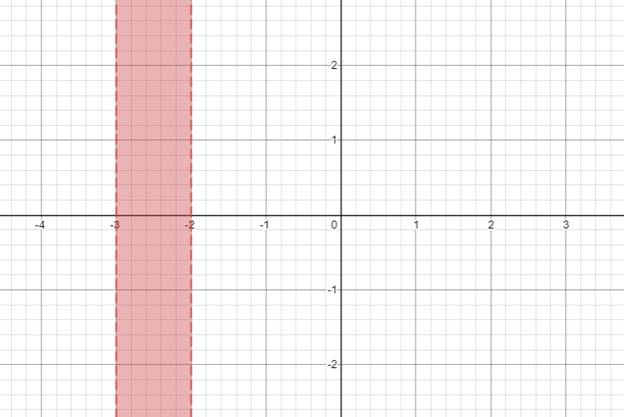# The solution of given nonlinear inequality equation and plot a graph for it.### Precalculus: Mathematics for Calcu...

6th Edition
Stewart + 5 others
Publisher: Cengage Learning
ISBN: 9780840068071### Precalculus: Mathematics for Calcu...

6th Edition
Stewart + 5 others
Publisher: Cengage Learning
ISBN: 9780840068071

#### Solutions

Chapter 1.7, Problem 49E
To determine

## The solution of given nonlinear inequality equation and plot a graph for it.

Expert Solution

x2,x1 and x3

### Explanation of Solution

Given:

Non-linear inequality equation

(x+2)(x1)(x3)0

Calculation,

Non-linear inequality equation,

(x+2)(x1)(x3)0Now,(x+2)0x2and(x1)0x1and(x3)0x3

Now, graph of (x+2)(x1)(x3)0Conclusion:

Hence, the values of x is x2,x1 and x3 .

### Have a homework question?

Subscribe to bartleby learn! Ask subject matter experts 30 homework questions each month. Plus, you’ll have access to millions of step-by-step textbook answers!